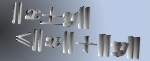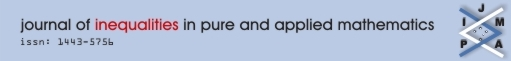Home Editors Submissions Reviews Volumes RGMIA About Us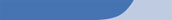Volume 8, Issue 2, Article 43

A New Subclass of \$k\$-Uniformly Convex Functions with Negative Coefficients

Authors: Hari M. Srivastava, T.N. Shanmugam, C. Ramachandran, S. Sivasubramanian,
Keywords: Analytic functions; Univalent functions; Coefficient inequalities and coefficient estimates; Starlike functions; Convex functions; Close-to-convex functions; \$k\$-Uniformly convex functions; \$k\$-Uniformly starlike functions; Uniformly starlike functions; Hadamard product (or convolution); Extreme points; Radii of close-to-convexity, starlikeness and convexity; Integral operators.
Date Accepted: 15/06/07
Subject Codes:

Primary 30C45.

Editors: Themistocles M. Rassias,

Abstract:

The main object of this paper is to introduce and investigate a subclass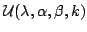of normalized analytic functions in the open unit disk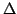, which generalizes the familiar class of uniformly convex functions. The various properties and characteristics for functions belonging to the classderived here include (for example) a characterization theorem, coefficient inequalities and coefficient estimates, a distortion theorem and a covering theorem, extreme points, and the radii of close-to-convexity, starlikeness and convexity. Relevant connections of the results, which are presented in this paper, with various known results are also considered.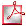Download Screen PDFDownload Print PDF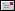Send this article to a friend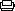Print this pagesearch [advanced search]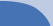copyright 2003terms and conditions login